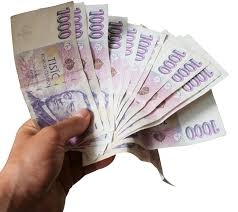# Two-thirds 7811

Mr. Trnka received a reward. He put half of it in the passbook, gave two-thirds of the rest to his wife, and still had € 1,200 left. How much did Mr. Trnka receive?

x =  7200

### Step-by-step explanation:Did you find an error or inaccuracy? Feel free to write us. Thank you!

Tips for related online calculators
Need help calculating sum, simplifying, or multiplying fractions? Try our fraction calculator.
Do you have a linear equation or system of equations and looking for its solution? Or do you have a quadratic equation?